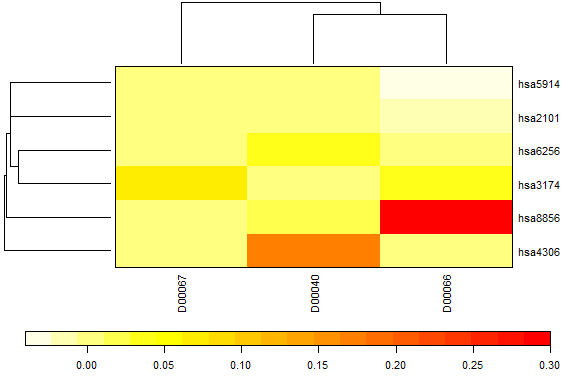# A short introduction to cross-network analysis with xnet

## Concepts and terms used in the package.

### Notation and naming of networks in the package

Networks exist in all forms and shapes. xnet is a simple, but powerful package to predict edges in networks in a supervised fashion. For example:

• which proteins interact with eachother?
• which goods are bought by which clients?
• how many likes give Twitter users to each other’s tweets?

The two sets can contain the same types nodes (e.g. protein interaction networks) or different nodes (e.g. goods bought by clients in a recommender system). When the two sets are the same, we call this a homogeneous network. A network between two different sets of nodes is called a heterogeneous network.

The interactions are presented in a adjacency matrix, noted Y. The rows of Y represent one set of nodes, the columns the second. Interactions can be measured on a continuous scale, indicating how strong each interaction is. Often the adjacency matrix only contains a few values: 1 for interaction, 0 for no interaction and possibly -1 for an inverse interaction.

Two-step kernel ridge regression ( function tskrr() ) predicts the values in the adjacency matrix based on similarities within the node sets, calculated by using some form of a kernel function. This function takes two nodes as input, and outputs a measure of similarity with specific mathematical properties. The resulting kernel matrix has to be positive definite for the method to work. In the package, these matrices are noted K for the rows and - if applicable - G for the columns of Y.

We refer to the kernlab for a collection of different kernel functions.

### Data in the package

For the illustrations, we use two different datasets.

#### Homogeneous networks

The example dataset proteinInteraction originates from a publication by Yamanishi et al (2004). It contains data on interaction between a subset of 769 proteins, and consists of two objects:

• the adjacency matrix proteinInteraction where 1 indicates an interaction between proteins
• the kernel matrix Kmat_y2h_sc describing the similarity between the proteins.

#### Heterogeneous networks

The dataset drugtarget serves as an example of a heterogeneous network and comes from a publication of Yamanishi et al (2008). In order to get a correct kernel matrix, we recalculated the kernel matrices as explained in the vignette Preparation of the example data.

The dataset exists of three objects

• the adjacency matrix drugTargetInteraction
• the kernel matrix for the targets targetSim
• the kernel matrix for the drugs drugSim

The adjacency matrix indicates which protein targets interact with which drugs, and the purpose is to predict new drug-target interactions.

## Fitting a two-step kernel ridge regression

### Heterogeneous network

To fit a two-step kernel ridge regression, you use the function tskrr(). This function needs to get some tuning parameter(s) lambda. You can choose to set 1 lambda for tuning K and G using the same lambda value, or you can specify a different lambda for K and G.


data(drugtarget)

drugmodel <- tskrr(y = drugTargetInteraction,
k = targetSim,
g = drugSim,
lambda = c(0.01,0.1))

drugmodel
#> Heterogeneous two-step kernel ridge regression
#> ---------------------------------------------
#> Dimensions: 26 x 54
#> Lambda:
#>    k    g
#> 0.01 0.10
#>
#> Row Labels:"hsa190" "hsa2099" "hsa2100" "hsa2101" "hsa2103" "hsa2104" ...
#> Col Labels:"D00040" "D00066" "D00067" "D00075" "D00088" "D00094" ...

### Homogeneous network

For homogeneous networks you use the same function, but you don’t specify the G matrix. You also need only a single lambda:

data(proteinInteraction)

proteinmodel <- tskrr(proteinInteraction,
k = Kmat_y2h_sc,
lambda = 0.01)

proteinmodel
#> Homogeneous two-step kernel ridge regression
#> -------------------------------------------
#> Dimensions: 150 x 150
#> Lambda:
#>    k
#> 0.01
#>
#> Labels:"YER171W" "YEL002C" "YJL210W" "YBR097W" "YHR174W" ...

### Extracting parameters from a trained model.

The model output itself tells you only little, apart from the dimensions, the lambdas used and the labels found in the data. That information can be extracted using a number of convenient functions.

lambda(drugmodel)  # extract lambda values
#>    k    g
#> 0.01 0.10
lambda(proteinmodel)
#>    k
#> 0.01
dim(drugmodel) # extract the dimensions
#>  26 54

protlabels <- labels(proteinmodel)
str(protlabels)
#> List of 2
#>  $k: chr [1:150] "YER171W" "YEL002C" "YJL210W" "YBR097W" ... #>$ g: chr [1:150] "YER171W" "YEL002C" "YJL210W" "YBR097W" ...
• lambda returns a vector with the lambda values used.
• dim returns the dimensions.
• labels returns a list with two elements, k and g, containing the labels for the rows resp. the columns.

You can also use the functions rownames() and colnames() to extract the labels.

### Information on the fit of the model

The functions fitted() and predict() can be used to extract the fitted values. The latter also allows you to specify new kernel matrices to predict for new nodes in the network. To obtain the residuals, you can use the function residuals(). This is shown further in the document.

## Performing leave-one-out cross-validation

### Settings for LOO

The most significant contribution of this package, are the various shortcuts for leave-one-out cross-validation (LOO-CV) described in the paper by Stock et al, 2018. Generally LOO-CV removes a value, refits the model and predicts the removed value based on this refit model. In this package you do this using the function loo(). The paper describes a number of different settings, which can be passed to the argument exclusion:

• interaction: in this setting only the interaction between two nodes is removed from the adjacency matrix.
• row: in this setting the entire row for that node is removed from the adjacency matrix. This boils down to removing a node from the set described by K.
• column: in this setting the entire column for that node is removed from the adjacency matrix. This boils down to removing a node from the set decribed by G.
• both: in this setting both rows and columns are removed, i.e. for every loo value the respective nodes are removed from both sets.

For some networks, only information of interactions is available, so a 0 does not necessarily indicate “no interaction”. It just indicates “no knowledge” for an interaction. In those cases it makes more sense to calculate the LOO values by replacing the interaction by 0 instead of removing it. This can be done by setting replaceby0 = TRUE.

loo_drugs_interaction <- loo(drugmodel, exclusion = "interaction",
replaceby0 = TRUE)
loo_protein_both <- loo(proteinmodel, exclusion = "both")

In both cases the result is a matrix with the LOO values.

### Use LOO in other functions

There are several functions that allow to use the LOO values instead of predictions for model tuning and validation. For example, you can calculate residuals based on LOO values directly using the function residuals():

loo_resid <- residuals(drugmodel, method = "loo",
exclusion = "interaction",
replaceby0 = TRUE)
all.equal(loo_resid,
response(drugmodel) - loo_drugs_interaction )
#>  TRUE

Every other function that can use LOO values instead of predictions will have the same two arguments exclusion and replaceby0.

## Looking at model output

The function provides a plot() function for looking at the model output. This function can show you the fitted values, LOO values or the residuals. It also lets you construct dendrograms based on distances computed using the K and G matrices, so you have both the predictions and the similarity information on the nodes in one plot.

plot(drugmodel, main = "Drug Target interaction")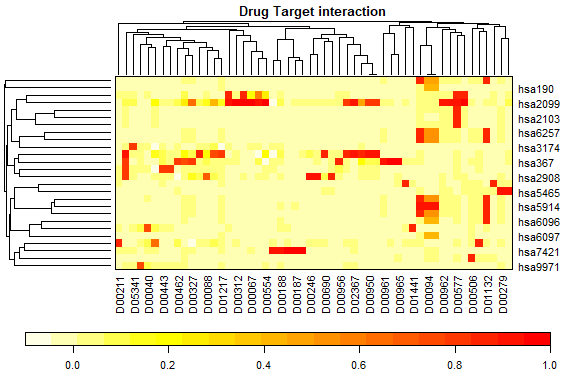To plot LOO values, you set the argument which. As the protein model is pretty extensive, we can remove the dendrogram and select a number of proteins we want to inspect closer.

plot(proteinmodel, dendro = "none", main = "Protein interaction - LOO",
which = "loo", exclusion = "both",
rows = rownames(proteinmodel)[10:20],
cols = colnames(proteinmodel)[30:35])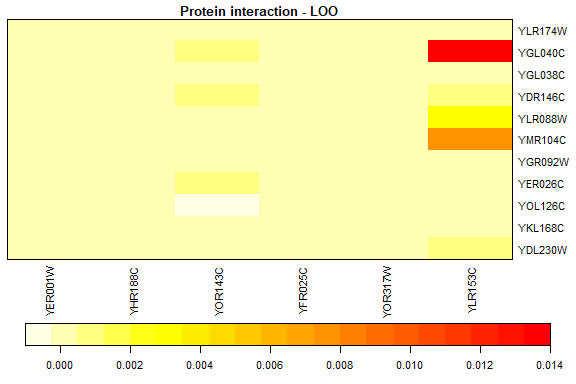If the colors don’t suit you, you can set both the breaks used for the color code and the color code itself.

plot(drugmodel, which = "residuals",
col = rainbow(20),
breaks = seq(-1,1,by=0.1))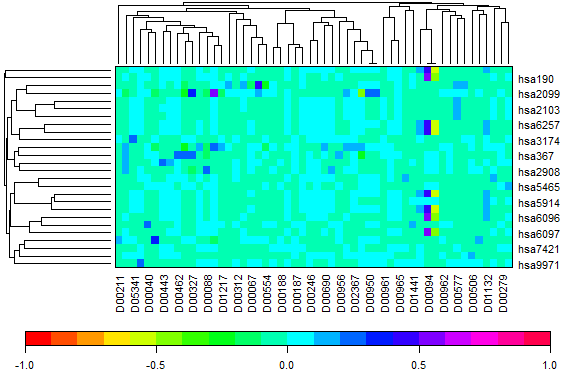## Tuning a model to find the best lambda.

In most cases you don’t know how to set the lambda values for optimal predictions. In order to find the best lambda values, the function tune() allows you to do a grid search. This grid search can be done in a number of ways:

• by specifying actual values to be tested
• by specifying the minimum and maximum lambda together with the number of values needed in every dimension. The function will create a grid that’s even spaced on a log scale.

Tuning minimizes a loss function. Two loss functions are provided, i.e. one based on mean squared error (loss_mse) and one based on the area under the curve (loss_auc). But you can provide your own loss function too, if needed.

### Homogeneous networks

Homogeneous networks have a single lambda value, and should hence only search in a single dimension. The following code tests 20 lambda values between 0.001 and 10.

proteintuned <- tune(proteinmodel,
lim = c(0.001,10),
ngrid = 20,
fun = loss_auc)
proteintuned
#> Tuned homogeneous two-step kernel ridge regression
#> -------------------------------------------------
#> Dimensions: 150 x 150
#> Lambda:
#>           k
#> 0.001623777
#>
#> Labels:"YER171W" "YEL002C" "YJL210W" "YBR097W" "YHR174W" ...
#>
#> Tuning information:
#> -------------------
#> exclusion setting: edges
#> loss value: 0.3294548
#> loss function: Area under curve (loss_auc)

The returned object is a again a model object with the model fitted using the best lambda value. It also contains extra information on the settings of the tuning. You can extract the grid values as follows:

get_grid(proteintuned)
#> $k #>  0.001000000 0.001623777 0.002636651 0.004281332 0.006951928 #>  0.011288379 0.018329807 0.029763514 0.048329302 0.078475997 #>  0.127427499 0.206913808 0.335981829 0.545559478 0.885866790 #>  1.438449888 2.335721469 3.792690191 6.158482111 10.000000000 This returns a list with one or two elements, each element containing the grid values for the respective kernel matrix. You can also create a plot to visually inspect the tuning: plot_grid(proteintuned)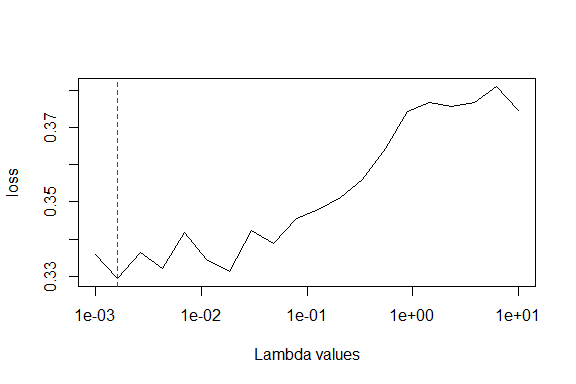This object is also a tskrr model, so all the functions used above can be used here as well. For example, we can use the same code as before to inspect the LOO values of this tuned model: plot(proteintuned, dendro = "none", main = "Protein interaction - LOO", which = "loo", exclusion = "both", rows = rownames(proteinmodel)[10:20], cols = colnames(proteinmodel)[30:35])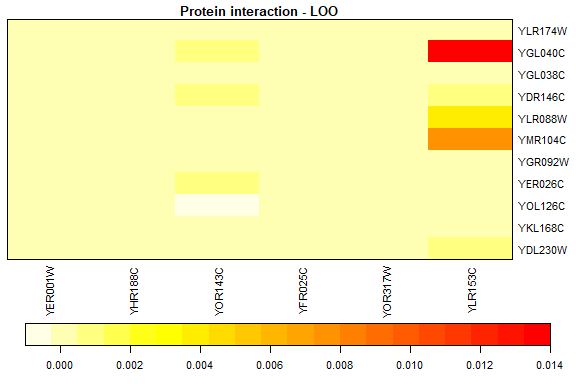### Heterogeneous networks For heterogeneous networks, the tuning works the same way. Standard, the function tune() performs a two-dimensional grid search. To do a one-dimensional grid search (i.e. use the same lambda for K and G), you set the argument onedim = TRUE. drugtuned1d <- tune(drugmodel, lim = c(0.001,10), ngrid = 20, fun = loss_auc, onedim = TRUE) plot_grid(drugtuned1d, main = "1D search")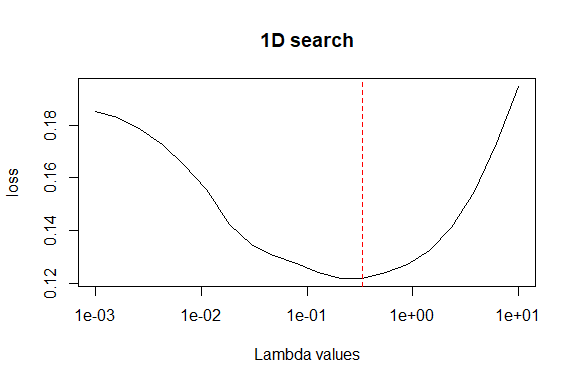When performing a two-dimensional grid search, you can specify different limits and grid values or lambda values for both dimensions. You do this by passing a list with two elements for the respective arguments. drugtuned2d <- tune(drugmodel, lim = list(k = c(0.001,10), g = c(0.0001,10)), ngrid = list(k = 20, g = 10), fun = loss_auc) the plot_grid() function will give you a heatmap indicating where the optimal lambda values are found: plot_grid(drugtuned2d, main = "2D search")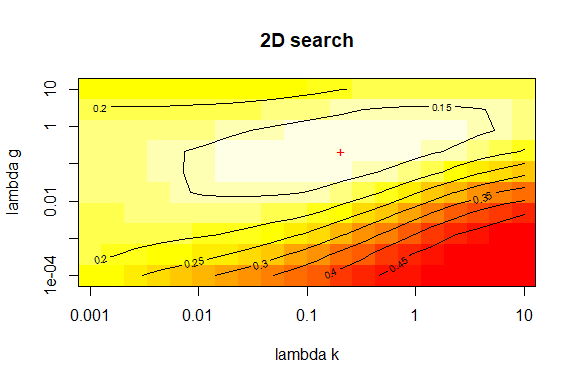As before, you can use the function lambda() to get to the best lambda values. lambda(drugtuned1d) #> k g #> 0.3359818 0.3359818 lambda(drugtuned2d) #> k g #> 0.2069138 0.2154435 A one-dimensional grid search give might yield quite different optimal lambda values. To get more information on the loss values, the function get_loss_values() can be used. This allows you to examine the actual improvement for every lambda value. The output is always a matrix, and in the case of a 1D search it’s a matrix with one column. Combining these values with the lambda grid, shows that the the difference between a lambda value of around 0.20 and around 0.34 is very small. This is also obvious from the grid plots shown above. cbind( loss = get_loss_values(drugtuned1d)[,1], lambda = get_grid(drugtuned1d)$k
)[10:15,]
#>           loss    lambda
#> [1,] 0.1275495 0.0784760
#> [2,] 0.1239134 0.1274275
#> [3,] 0.1219094 0.2069138
#> [4,] 0.1217656 0.3359818
#> [5,] 0.1238880 0.5455595
#> [6,] 0.1271689 0.8858668

## Predicting new values

In order to predict new values, you need information on the outcome of the kernel functions for the combination of the new values and those used to train the model. Depending on which information you have, you can do different predictions. To illustrate this, we split up the data for the drugsmodel.

idk_test <- c(5,10,15,20,25)
idg_test <- c(2,4,6,8,10)

drugInteraction_train <- drugTargetInteraction[-idk_test, -idg_test]
target_train <- targetSim[-idk_test, -idk_test]
drug_train <- drugSim[-idg_test, -idg_test]

target_test <- targetSim[idk_test, -idk_test]
drug_test <- drugSim[idg_test, -idg_test]

So the following drugs and targets are removed from the training data and will be used for predictions later:

rownames(target_test)
#>  "hsa2103" "hsa4306" "hsa5915" "hsa6256" "hsa9970"
colnames(drug_test)
#>   "D00040" "D00067" "D00088" "D00105" "D00143" "D00182" "D00187" "D00188"
#>   "D00211" "D00246" "D00279" "D00299" "D00312" "D00316" "D00327" "D00348"
#>  "D00443" "D00462" "D00506" "D00554" "D00565" "D00577" "D00585" "D00586"
#>  "D00596" "D00627" "D00690" "D00730" "D00898" "D00930" "D00950" "D00951"
#>  "D00954" "D00956" "D00961" "D00962" "D00965" "D01115" "D01132" "D01161"
#>  "D01217" "D01294" "D01387" "D01441" "D01689" "D02217" "D02367" "D04066"
#>  "D05341"

We can now train the data using tune() just like we would use tskrr()

trained <- tune(drugInteraction_train,
k = target_train,
g = drug_train,
ngrid = 30)

### Predict for new K-nodes

In order to predict the interaction between new targets and the drugs in the model, we need to pass the kernel values for the similarities between the new targets and the ones in the model. The predict() function will select the correct G matrix for calculating the predictions.

Newtargets <- predict(trained, k = target_test)
Newtargets[, 1:5]
#>              D00040     D00067      D00088     D00105      D00143
#> hsa2103 0.004057079 0.03081055 0.009556427 0.04742997 0.008212541
#> hsa4306 0.001703995 0.02759991 0.175792592 0.04809118 0.024805465
#> hsa5915 0.023949572 0.01421044 0.008865099 0.01646451 0.016024808
#> hsa6256 0.010536788 0.02970235 0.018628412 0.03806541 0.003662778
#> hsa9970 0.015759320 0.02500044 0.035086840 0.04464471 0.181819822

### Predict for new G-nodes

If you want to predict for new drugs, you need the kernel values for the similarities between new drugs and the drugs trained in the model.

Newdrugs <- predict(trained, g = drug_test)
Newdrugs[1:5, ]
#>                D00066       D00075       D00094        D00129        D00163
#> hsa190   0.0004385072 3.910979e-05 0.0000317464  5.801116e-04  0.0003651196
#> hsa2099  0.0268199330 3.264114e-01 0.0227098621 -1.072246e-01  0.0623070092
#> hsa2100 -0.0289764612 3.207540e-01 0.0147790518 -1.264217e-01 -0.1383998327
#> hsa2101 -0.0048777886 1.309579e-02 0.0090779102  4.106452e-06 -0.0593089366
#> hsa2104 -0.0051459974 1.258277e-02 0.0095775683  1.577701e-05 -0.0596787553

### Predict for new K and G nodes

You can combine both kernel matrices used above to get predictions about the interaction between new drugs and new targets:

Newdrugtarget <- predict(trained, k=target_test, g=drug_test)
Newdrugtarget
#>                D00066     D00075       D00094       D00129        D00163
#> hsa2103  7.715195e-03 0.02921056 -0.012015102  0.008404732 -4.006168e-02
#> hsa4306  1.134892e-01 0.13809671  0.002077053 -0.024120960  2.336087e-02
#> hsa5915 -1.173301e-05 0.02535359  0.423718351  0.012963952 -1.475475e-02
#> hsa6256  2.490618e-02 0.03032823  0.063798137  0.005722959  5.630761e-05
#> hsa9970  5.919073e-02 0.02541274  0.031471514  0.135712388  5.288650e-02

## Impute new values based on a tskrr model

Sometimes you have missing values in a adjacency matrix. These missing values can be imputed based on a simple algorithm:

1. replace the missing values by a start value
2. fit a tskrr model with the added values
3. replace the missing values with the predictions of that model
4. repeat until the imputed values converge (i.e. the difference with the previous run falls below a tolerance value)

Apart from the usual arguments of tskrr, you can give additional parameters to the function impute_tskrr. The most important ones are

• niter: the maximum number of iterations
• tol: the tolerance, i.e. the minimal sum of squared differences between iteration steps to keep the algorithm going
• verbose: setting this to 1 or 2 gives additional info on the algorithm performance.

So let’s construct a dataset with missing values:

drugTargetMissing <- drugTargetInteraction
idmissing <- c(10,20,30,40,50,60)
drugTargetMissing[idmissing] <- NA

Now we can try to impute these values. The outcome is again a tskrr model.

imputed <- impute_tskrr(drugTargetMissing,
k = targetSim,
g = drugSim,
verbose = TRUE)
#> Nr. of iterations: 80 - Deviation:1.4900616992948e-08
plot(imputed, dendro = "none")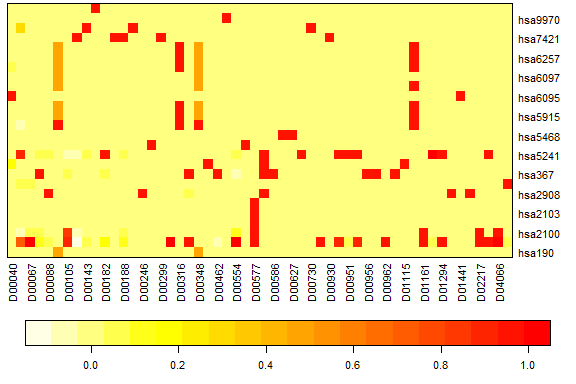To extract information on the imputation, you have a few convenience functions to your disposal:

• has_imputed_values() tells you whether the model contains imputed values
• is_imputed() returns a logical matrix where TRUE indicates an imputed value
• which_imputed() returns an integer vector with the positions of the imputed values. Note that these positions are vector positions, i.e. they give the position in a single dimension (according to how a matrix is stored internally in R.)
has_imputed_values(imputed)
#>  TRUE
which_imputed(imputed)
#>  10 20 30 40 50 60

# Extract only the imputed values
id <- is_imputed(imputed)
predict(imputed)[id]
#>   0.17454499  0.03111647 -0.01402729 -0.03812844  0.28626726  0.06590431

You can use this information to plot the imputed values in context:

rowid <- rowSums(id) > 0
colid <- colSums(id) > 0
plot(imputed, rows = rowid, cols = colid)